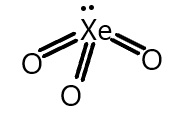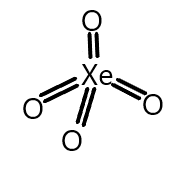QuestionAnswers

# What would be the number of $p\pi - d\pi$ ‘pi’ bonds present in $Xe{O_3}$ and $Xe{O_4}$ molecules?A) $3,4$B) $4,2$C) $2,3$D) $3,2$Verified
91.8k+ views
Hint: As we know that Xenon hexafluoride on hydrolysis with water gives Xenon trioxide and from their structures we can calculate the number of sigma and pi-bonds in a molecules where a double bond contains one sigma and one pi-bond and a triple bond contains one sigma and two pi-bonds.

We know that Xenon trioxide is formed on hydrolysis of xenon hexafluoride and the electronic configuration of xenon is $n{s^2}n{p^6}$ where d-orbital is vacant and thus involved in the hybridisation and the structure of xenon trioxide can be seen as below:We also know that sigma and pi-bonds are formed by overlapping atomic orbitals. End to end overlapping of atomic orbitals results in sigma bonds formation and pi bond is formed by sideways overlapping of atomic orbitals. And the participation of d-orbital in hybridisation can take place when d-orbitals are lying vacant or partially filled.
Similarly, in the structure of xenon trioxide, it has three $p\pi - d\pi$ bonds which correspond to the p-orbital from the oxygen atoms and the d-orbital is from the xenon atom.
Similarly, the structure of xenon tetroxide is given and the electronic configuration is again the same and it also possesses a vacant d-orbital. So the structure is given as:We can see that xenon tetroxide contains four $p\pi - d\pi$ bonds where p-orbital is again from oxygen and d-orbital is from xenon atom.
Hence, from the above explanation we can see that $Xe{O_3}$ and $Xe{O_4}$ possess $3,4$$p\pi - d\pi$ pi bonds.
Therefore, the correct answer is (A).

Note: Always remember that the xenon trioxide,$Xe{O_3}$ is a colourless explosive solid and has a pyramidal molecular structure and the xenon tetroxide,$Xe{O_4}$ possess a tetrahedral structure both and both these molecules contains a total of three and four $p\pi - d\pi$ pi-bonds respectively.